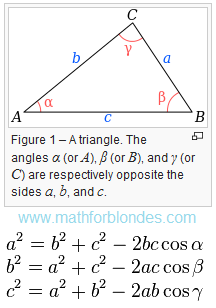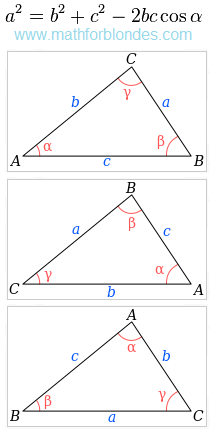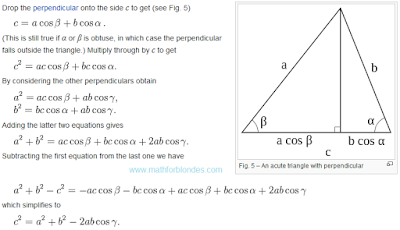## 3/13/2016

### Law of cosines

The triangle has three sides and three corners. Appearance cosine theorem depends on the received angles and sides of the triangle symbols. Here's how it looks in Wikipedia.Law of cosines

Three angle of the triangle gives three options for the formula of one triangle. In law of cosines can use a one formula, and three variants of symbols.Three variants of symbols

These two options allow to describe all sides and angles of the triangle. The traditional problems of mathematics we are taught to find one of the triangle elements.

Question: Can one formula with one variant of symbols to describe all the elements of the triangle?

Here's how to do it using the cosine theorem.

The proof of the theorem of cosines in the trigonometric form looks like.The proof using trigonometry

If you change the "minus" sign in the "plus" sign, we get the cosine theorem for the perimeter of the triangle.

Law of cosines in general form (in Russian).